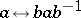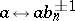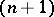# Markov braid theorem

(diff) ← Older revision | Latest revision (diff) | Newer revision → (diff)

If two closed braids represent the same ambient isotopy class of oriented links (cf. also Braid theory), then one can transform one braid to another by a sequence of Markov moves:

i)(conjugation).

ii), whereis an element of theth braid groupandis theth generator of theth braid group.

Markov's braid theorem is an important ingredient in the construction of the Jones polynomial and its generalizations (e.g. the Jones–Conway polynomial).

How to Cite This Entry:
Markov braid theorem. Encyclopedia of Mathematics. URL: http://encyclopediaofmath.org/index.php?title=Markov_braid_theorem&oldid=17995
This article was adapted from an original article by Jozef Przytycki (originator), which appeared in Encyclopedia of Mathematics - ISBN 1402006098. See original article